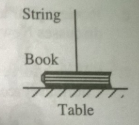# Problem: 1. A string is tied to a book and pulled lightly as shown in the diagram. The book remains in contact with the table and does not move. a. Draw a free-body diagram for the book. Label each of the forces exerted on the book as you were instructed in the tutorial Forces. b. How do the forces exerted on the book, in this case, compare to the forces exerted on the book when the string is not present?

###### FREE Expert Solution

In free body diagrams, we draw a dot to represent the object.

All forces are represented by vectors. The tail of the vectors touches the dot.

(a)

The tension, T, in the string points up.

The weight, w, of the book is directed downward.

82% (7 ratings)###### Problem Details

1. A string is tied to a book and pulled lightly as shown in the diagram. The book remains in contact with the table and does not move.a. Draw a free-body diagram for the book. Label each of the forces exerted on the book as you were instructed in the tutorial Forces.

b. How do the forces exerted on the book, in this case, compare to the forces exerted on the book when the string is not present?